5. Mathematical Structure of Elementary Variable Judgements of Dialectical Logic

L. G. Kreidik

5.1. The fundamental frequency

Having got acquainted with manifestations of the fundamental period, we will go back to logic of judgements. An elementary dialectical material-ideal judgement concerned with quantitative and qualitative sides of an objective process, recurring on the ideal side and nonrecurring on the material one, with one variable relative argument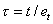, where t is an absolute argument and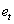is a unit measure of the absolute argument, has the form (see also (1.79)):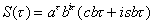. (1.93)

Norms of material and ideal periods of the judgement are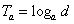,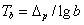. (1.94)

If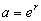and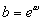are assumed, then (1.93) can write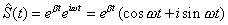,

where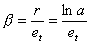,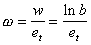and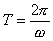. (1.95)

The material period with a decimal scale is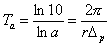. (1.96)

If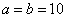, then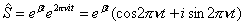, (1.97)

where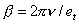and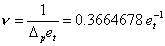is the fundamental frequency.

5.2. The harmonic unit of a judgement

The periodic component of the oppositus (1.97) is a variable quantitative-qualitative harmonic unit of a judgement, which will be denoted by: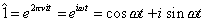. (1.98)

Then the contradictory oppositus of the modulus a can be written as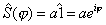or, where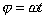. (1.99)

The opposite is uniquely defined by the harmonic unit but its meaning is ambiguous as it can describe a unit wave of affirmation-negation, a rotating quantitative unit, the helix of motion, etc.

Every state of the unit recurs many times and therefore it is necessary that qualitative changes in the unit be taken into consideration. As different states of the harmonic unit, units of affirmation are described by the discrete equation, (1.100)

where n is the order of the unit.

As quantitative units, all the units are equal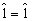, but as qualitative units, they are different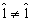. Since the quantitative and qualitative sides of the unit are inseparable, equality of the units on the quantitative side q and inequality of the units on the qualitative side k occurs simultaneously: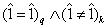. (1.101)

This is in full agreement with the "Yes-No" formula of dialectical logic. Equality-inequality (1.101) is consistent with the objective state of affairs and therefore it is true. In the case of two different states of the unit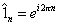and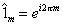, (1.102)

we have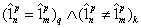, if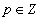. (1.103)

When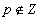, then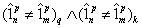(1.104)

This relation corresponds to the "No - No" dialectical formula.

5.3. A dialectical quantitative-qualitative numerical field and a Geld of complex numbers

In the field of complex numbers many functions are multi-valued, whereas dialectical oppositi of the same design are unique. This difference is in principle. For example, the fc-th root of unity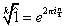(1.105)

is unique as one and only one root corresponds to every unity in the state n and different roots correspond to different states, for example, n and m.

The dialectical quantitative-qualitative numerical field is similar by form to the field of complex numbers. However, they are different fields and formal extension of the notions, axioms, and theorems of one number set to another is inadmissible.

The fundamentals of classical mathematics are constructed following the laws of formal logic, rejecting any contradictions, including the true ones. For more than two thousands years, classical mathematics has been trying to construct noncontradictory theories that cannot be created in principle. Of course, the presence of absurd contradictions in a theory is inadmissible and in this sense any theory should be noncontradictory. This does not mean, however, that it should not contain correct, dialectical contradictions. Moreover, if a theory does not contain dialectical contradictions, it is approximate and false to some extent. It is necessary to bear in mind the undoubted fact that the World is dialectical and we should speak with It by the language of dialectical logic, the language of contradictions-noncontradictions.

Theoretical Dialectical Journal: Physics-Mathematics-Logic-Philosophy, N.1, site http://www.matdial.narod.ru/